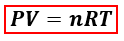# Problem: The following equation shows the combustion of methane.CH4 (g) + 2O2(g) → CO2(g) + 2H2O(g)If 6.0 g of CH4 reacts completely, what is the final pressure of the CO 2 produced and collected in a 1.4 L container at a temperature of 21°C?

🤓 Based on our data, we think this question is relevant for Professor Gellene's class at TTU.

###### FREE Expert Solution

We are asked to calculate the final pressure of CO2 produced from the combustion of CH4. We can calculate the pressure of the gas using the ideal gas equation:P = pressure, atm
V = volume, L
n = moles, mol
R = gas constant = 0.08206 (L·atm)/(mol·K)
T = temperature, K

In the ideal gas equation, the amount of CO2 in moles is needed but the mass of the CH4 is given instead. We will have to calculate the moles of CO2 produced from CH4 first using the balanced reaction equation and mole to mole comparison.###### Problem Details

The following equation shows the combustion of methane.

CH4 (g) + 2O2(g) → CO2(g) + 2H2O(g)

If 6.0 g of CH4 reacts completely, what is the final pressure of the CO 2 produced and collected in a 1.4 L container at a temperature of 21°C?